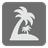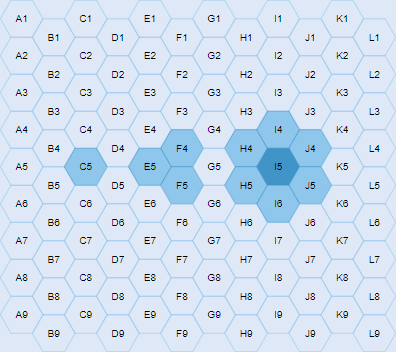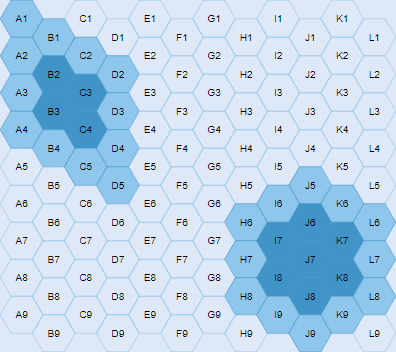Hexagonal Islands
Simple+
English

This is the mission similar to the “Calculate Islands” mission where you’re dealing with a hexagonal grid. Please, help the robots again.

The size of the hexagonal grid is 12x9 ('A1' to 'L9').

• It’s characteristics:
• the alphabet letters represent columns and numbers represent rows;
• it’s flat topped;
• the even columns are being shoved down.

You are given a set of coastal hexes for all islands as input value. You have to find all islands that those hexes represent and return a list (tuple or iterable) of that size.

Note:

• The islands don't include other islands.
• If there is no island (input == set ()), return [].
• There are also case that no sea. (In this case your answer is .)

Examples:

```sorted(hexagonal_islands({'C5','E5','F4','F5','H4','H5','I4','I6','J4','J5'})) == [1, 3, 7]
sorted(hexagonal_islands({'A1','A2','A3','A4','B1','B4','C2','C5','D2', 'D3','D4','D5',
'H6','H7','H8','I6','I9','J5','J9','K6','K9','L6','L7','L8'}) == [16, 19]
sorted(hexagonal_islands(set())) == []
```Input: The coastal hexes of all islands (a set of strings).

Output: The sizes of all islands (an iterable ( a list or tuple) of integers).

How it is used:

For recognizing the area on the hexagonal grid.

Preconditions:

• all(re.fullmatch('[A-L][1-9]', i) for i in input)